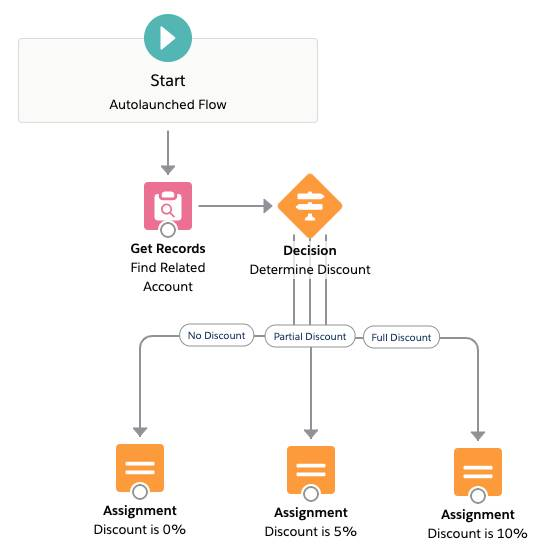Empiece a realizar un seguimiento de su progreso
Estimación de tiempo

# Build Logic to Determine the Right Discount to Apply

## Determine the Right Discount to Apply

Next, the flow needs to determine the discount to apply. In their new discounting program, Acme Wireless awards discounts based on the account’s annual revenue: the higher the revenue, the larger the discount. In this step, a Decision element routes the flow along one of several paths—also known as outcomes—depending on annual revenue.

Create three outcomes based on annual revenue.

• Full Discount Applied when the account’s annual revenue is greater than \$200,000,000
• Partial Discount Applied when the account’s annual revenue is between \$100,000,000 and \$200,000,000
• No Discount (default) Applied when neither of the other outcomes’ conditions are met
1. From the Elements tab, click and drag a Decision element onto the canvas.
2. For Label, enter Determine Discount.
3. Create an outcome for the full discount.
1. Under OUTCOME DETAILS, for Label, enter Full Discount.
2. Set the outcome criteria. Type Account in Resource, click on Account from Find_Related_Account >, then select AnnualRevenue.
Field Value
Resource {!Find_Related_Account.AnnualRevenue}
Operator Greater Than
Value 200000000
These criteria tell the element to route the flow along the Full Discount path only if the annual revenue of the account is greater than \$200,000,000.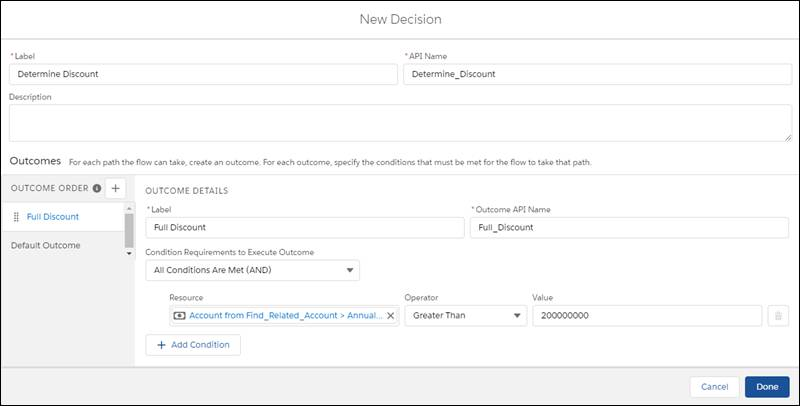• Create an outcome for the partial discount. This outcome is based on the annual revenue being between two values, so use two criteria.
1. Click + by OUTCOME ORDER.
2. For Label, enter Partial Discount.
3. Set the outcome criteria for the lower revenue limit.
Field Value
Resource {!Find_Related_Account.AnnualRevenue}
Operator Greater Than or Equal
Value 100000000
4. Click Add Condition. When you add a condition, Flow Builder applies the boolean operator AND by default. If you want the flow to take this path, every condition must be true. Here, AND is exactly what you want: only apply a partial discount when the annual revenue is above the lower revenue limit AND below the upper revenue limit.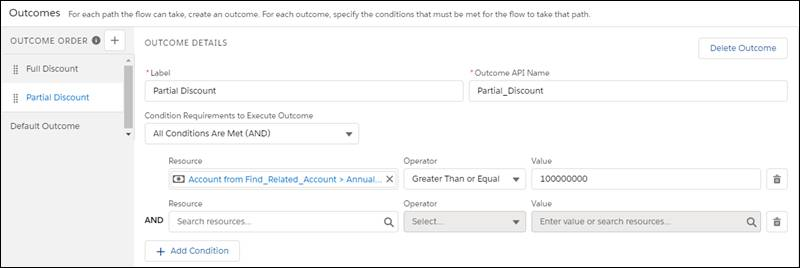5. Set the outcome criteria for the upper revenue limit.
Field Value
Resource {!Find_Related_Account.AnnualRevenue}
Operator Less Than or Equal
Value 200000000
These criteria tell the element to route the flow along the partial discount path only if the annual revenue of the account is greater than or equal to \$100,000,000 and less than or equal to \$200,000,000.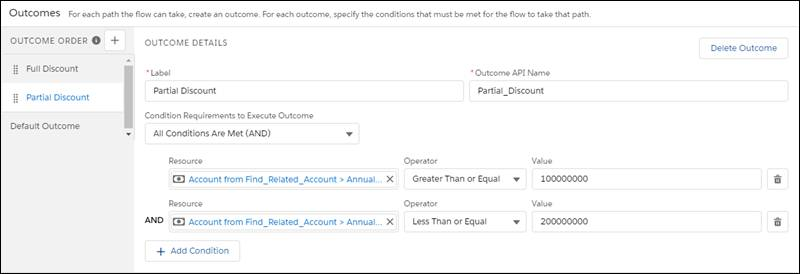• Configure the default outcome, which occurs if the account’s annual revenue doesn’t meet the criteria for a full discount or a partial discount.
1. Click [Default Outcome].
2. For Label, replace the predefined value with No Discount.
3. Click Done.
• Drag the node from the bottom of Start onto Find Related Account.
• Drag the node from the bottom of Find Related Account onto Determine Discount.
• Just to be safe, save your progress. Continue to ignore the warnings.
Here’s what your flow should look like.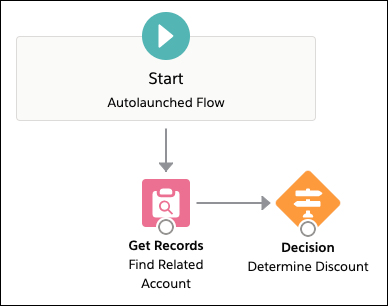## Assign Numerical Values to Discounts

At this point, the flow knows the annual revenue of the account and can use that value to determine the right kind of discount. But the discounts—full, partial, or no—are abstract. To be useful, each discount needs to link to a numerical value: 10 percent, 20 percent, and so forth.

Here, you’ll create a variable in which to store the discount values. Then you’ll use Assignment elements to update that variable with the right percentage based on the outcome: full, partial, or none. To change a variable’s value, you’ll use the Assignment element’s Equals operator. It sets a variable to a value, such as a number or string.

## Full Discount

1. From the Elements tab, click and drag an Assignment element onto the canvas.
2. For Label, enter Discount is 10%.
3. To store the discount percentage, create a variable. You’ll also use this variable for the percentages of the other outcomes.
1. For Variable, select New Resource.
2. Configure the variable.
Field Value
Resource Type Variable
API Name Discount
Description Stores the discount percentage in whole number format
Data Type Number
Decimal Places 2
Available for input and Available for output Leave unchecked
3. Click Done. You’ll use this variable to update the opportunity later. Just like the name variable suggests, its value varies based on which discount the opportunity gets.
4. For Operator, select Equals.
5. For Value, enter 10.6. Click Done.
7. Drag the node from the bottom of Determine Discount onto Discount is 10%.
8. Verify that the Outcome is set to Full Discount and click Done.Here’s what your flow should look like.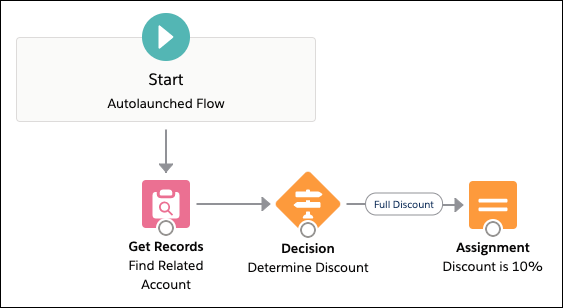## Partial Discount

A full discount isn’t the only possible outcome in this flow, so let’s configure another assignment—this one for when a partial discount is appropriate.
1. From the Elements tab, click and drag an Assignment element onto the canvas.
2. For Label, enter Discount is 5%.
3. For Variable, select Discount.
4. For Operator, select Equals.
5. For Value, enter 5.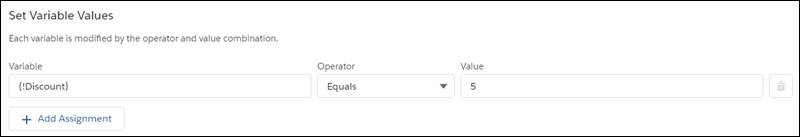6. Click Done.
7. Drag the node from the bottom of Determine Discount onto Discount is 5%.
8. Verify that the Outcome is set to Partial Discount and click Done.Here’s what your flow should look like.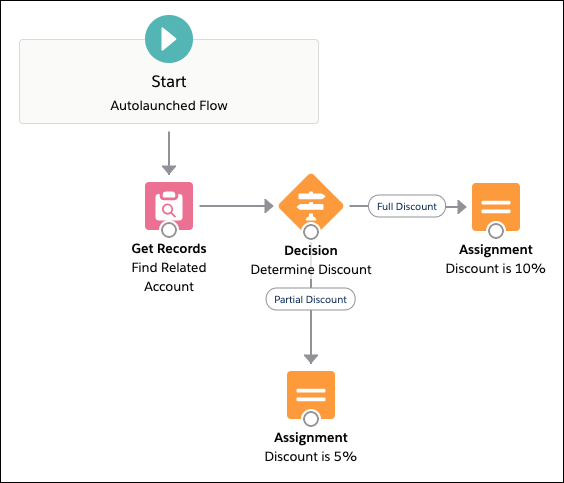## No Discount

One last outcome to take care of! You need an Assignment element to cover the scenario where the opportunity isn’t eligible for a discount.
1. From the Elements tab, click and drag an Assignment element onto the canvas.
2. For Label, enter Discount is 0%.
3. For Variable, select Discount.
4. For Operator, select Equals.
5. For Value, enter 0. Here's what the Set Variable Values overlay should look like.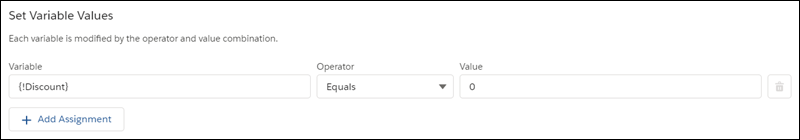6. Click Done.
7. Drag the node from the bottom of Determine Discount onto Discount is 0%. Because you've already connected the Full and Partial Discount outcomes, Flow Builder assigns the only remaining outcome—No Discount—to this path automatically.
8. Just to be safe, save the flow. Continue to ignore the warnings.
Here’s what your flow should look like.